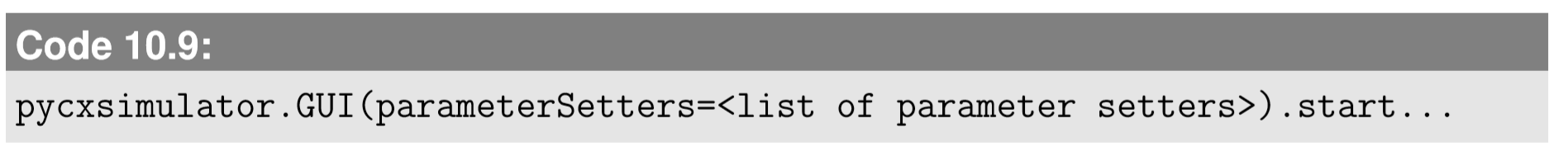$$\newcommand{\id}{\mathrm{id}}$$ $$\newcommand{\Span}{\mathrm{span}}$$ $$\newcommand{\kernel}{\mathrm{null}\,}$$ $$\newcommand{\range}{\mathrm{range}\,}$$ $$\newcommand{\RealPart}{\mathrm{Re}}$$ $$\newcommand{\ImaginaryPart}{\mathrm{Im}}$$ $$\newcommand{\Argument}{\mathrm{Arg}}$$ $$\newcommand{\norm}{\| #1 \|}$$ $$\newcommand{\inner}{\langle #1, #2 \rangle}$$ $$\newcommand{\Span}{\mathrm{span}}$$

# 10.3: Interactive Parameter Control in PyCX

$$\newcommand{\vecs}{\overset { \rightharpoonup} {\mathbf{#1}} }$$ $$\newcommand{\vecd}{\overset{-\!-\!\rightharpoonup}{\vphantom{a}\smash {#1}}}$$$$\newcommand{\id}{\mathrm{id}}$$ $$\newcommand{\Span}{\mathrm{span}}$$ $$\newcommand{\kernel}{\mathrm{null}\,}$$ $$\newcommand{\range}{\mathrm{range}\,}$$ $$\newcommand{\RealPart}{\mathrm{Re}}$$ $$\newcommand{\ImaginaryPart}{\mathrm{Im}}$$ $$\newcommand{\Argument}{\mathrm{Arg}}$$ $$\newcommand{\norm}{\| #1 \|}$$ $$\newcommand{\inner}{\langle #1, #2 \rangle}$$ $$\newcommand{\Span}{\mathrm{span}}$$ $$\newcommand{\id}{\mathrm{id}}$$ $$\newcommand{\Span}{\mathrm{span}}$$ $$\newcommand{\kernel}{\mathrm{null}\,}$$ $$\newcommand{\range}{\mathrm{range}\,}$$ $$\newcommand{\RealPart}{\mathrm{Re}}$$ $$\newcommand{\ImaginaryPart}{\mathrm{Im}}$$ $$\newcommand{\Argument}{\mathrm{Arg}}$$ $$\newcommand{\norm}{\| #1 \|}$$ $$\newcommand{\inner}{\langle #1, #2 \rangle}$$ $$\newcommand{\Span}{\mathrm{span}}$$

In Code 10.6, the parameter values are all directly given in the code and are not change able from the control window. PyCX has a feature, however,by which you can create interactive parameter setters (thanks to Przemysław Szufel and Bogumił Kami´nski at the Warsaw School of Economics who developed this nice feature!). A parameter setter should be deﬁned as a function in the following format:This may look a bit confusing, but all you need to do is to ﬁll in the <...> parts. Note that the int function in the code above may need to be changed according to the type of the parameter (float for real-valued parameters, str for string-valued ones, etc.). Once you deﬁne your own parameter setter functions, you can include them as an option when you call the pycxsimulator.GUI() function as follows:Here is an example of a parameter setter implementation that allows you to interactively change the number of particles:Once you apply this modiﬁcation to Code 10.6 and run it, the new parameter setter appears under the “Parameters” tab of the control window (Fig. 10.4.1). You can enter a new value into the input box, and then click either “Save parameters to the running model” or “Save parameters to the model and reset the model,” and the new parameter value is reﬂected in the model immediately.

Exercise $$\PageIndex{1}$$

To the code developed above, add one more parameter setter for sd (standard deviation of Gaussian noise for random motion of particles).